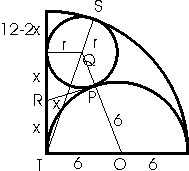Mathematical and Physical Journal
for High Schools
Issued by the MATFUND Foundation
 Already signed up? New to KöMaL?

#Problem C. 872. (November 2006)

C. 872. Consider a quadrant of a disc of radius 12 cm. A semicircle is drawn over one of the radii bounding the quadrant, and cut out of it. What is the radius of the largest possible circle that can be inscribed in the remaining figure?

(5 pont)

Deadline expired on December 15, 2006.

Sorry, the solution is available only in Hungarian. Google translation

Megoldás. A PQR derékszögű háromszögben RQ2=r2+x2, a POR derékszögű háromszögben RO2=62+x2. Az OQR derékszögű háromszögben RQ2+RO2=QO2, vagyis r2+x2+62+x2=(r+6)2, amiből 2x2=12r.

ST=SQ+QT, vagyis, amit rendezve, négyzetre emelve és rendezve r=3.### Statistics:

 393 students sent a solution. 5 points: 319 students. 4 points: 12 students. 3 points: 5 students. 2 points: 3 students. 1 point: 3 students. 0 point: 51 students.

Problems in Mathematics of KöMaL, November 2006